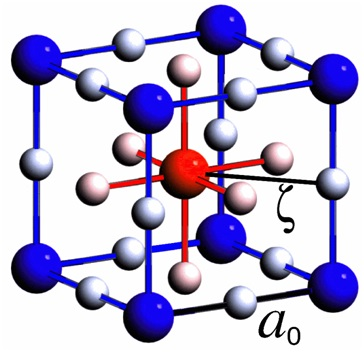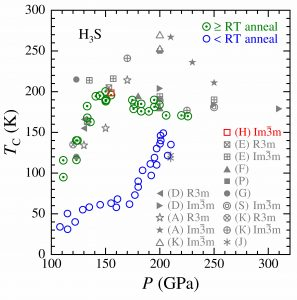# Compressed H3S: Inter-Sublattice Coulomb Coupling in a High-Tc Superconductor

Compressed H3S: inter-sublattice Coulomb coupling in a high-TC superconductor, D. R. Harshman and A. T. Fiory [arXiv]

Upon thermal annealing at or above room temperature (RT) and at high hydrostatic pressure P ~ 155 GPa, sulfur trihydride H3S exhibits a measured maximum superconducting transition temperature T­C ~ 200 K.  Various theoretical frameworks incorporating strong electron-phonon coupling and Coulomb repulsion have reproduced this record-level TC.  Of particular relevance is that experimentally observed H-D isotopic correlations among TC, P, and annealed order indicate an H-D isotope effect exponent α limited to values ≤ 0.183, leaving open for consideration unconventional high-TC superconductivity with electronic-based enhancements.  The work presented herein examines Coulombic pairing arising from interactions between neighboring S and H species on separate interlaced sublattices constituting H3S in the Im3m structure.  The optimal value of the transition temperature is calculated from TC0 = kB–1Λe2/ℓζ, with Λ= 0.007465 Å, inter-sublattice S-H separation spacing ζ = a0/2, interaction charge linear spacing ℓ = a0 (3/σ)1/2, average participating charge fraction σ = 3.43 ± 0.10 estimated from calculated H-projected electron states, and lattice parameter a0 = 3.0823 Å at P = 155 GPa.  The resulting value of TC0 = 198.5 ± 3.0 K is in excellent agreement with transition temperatures determined from resistivity (196 – 200 K onsets, 190 – 197 K midpoints), susceptibility (200 K onset), and critical magnetic fields (203.5 K by extrapolation).  Analysis of mid-infrared reflectivity data confirms the expected correlation between boson energy and ζ–1. Suppression of TC below TC0, correlating with increasing residual resistance for < RT annealing, is treated in terms of scattering-induced pair breaking. Correspondences between H3S and layered high-TC superconductor structures are also discussed, and a model considering Compton scattering of virtual photons of energies ≤ e2/ζ by inter-sublattice electrons is introduced, illustrating that Λ ∝ ƛC, where ƛC is the reduced electron Compton wavelength.Illustration of Im3m unit cell of compressed H3S with color contrast distinguishing the two simple cubic sublattices; basis H3S is shown with S larger than H. Lattice parameter a0 is cube edge; inter-sublattice S-H distance ζ is one-half cube face diagonal. Variation of measured TC of H3S with applied pressure P for ≥ RT (room temperature) anneal, green circles with center dot, and < RT anneal, blue circles (from [1, 5, 6]). Gray symbols are various theoretical calculations denoted as (D) , (E) , (P) , (A) , (K) , (G) , (F) , (S) , (К) , and (J) ; structure indicated where available. Red square symbol (H) corresponds to TC0 from this work.Dale R. Harshman and Anthony T. Fiory, Journal of Physics: Condensed Matter  29, 445702 (2017).